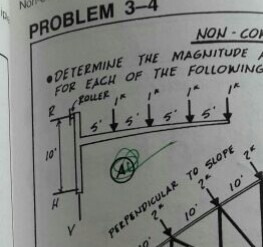# help..### Re: help..

What is the problem here. The figure is not enough for us to understand what is required?

• Mathematics inside the configured delimiters is rendered by MathJax. The default math delimiters are $$...$$ and $...$ for displayed mathematics, and $...$ and $...$ for in-line mathematics.